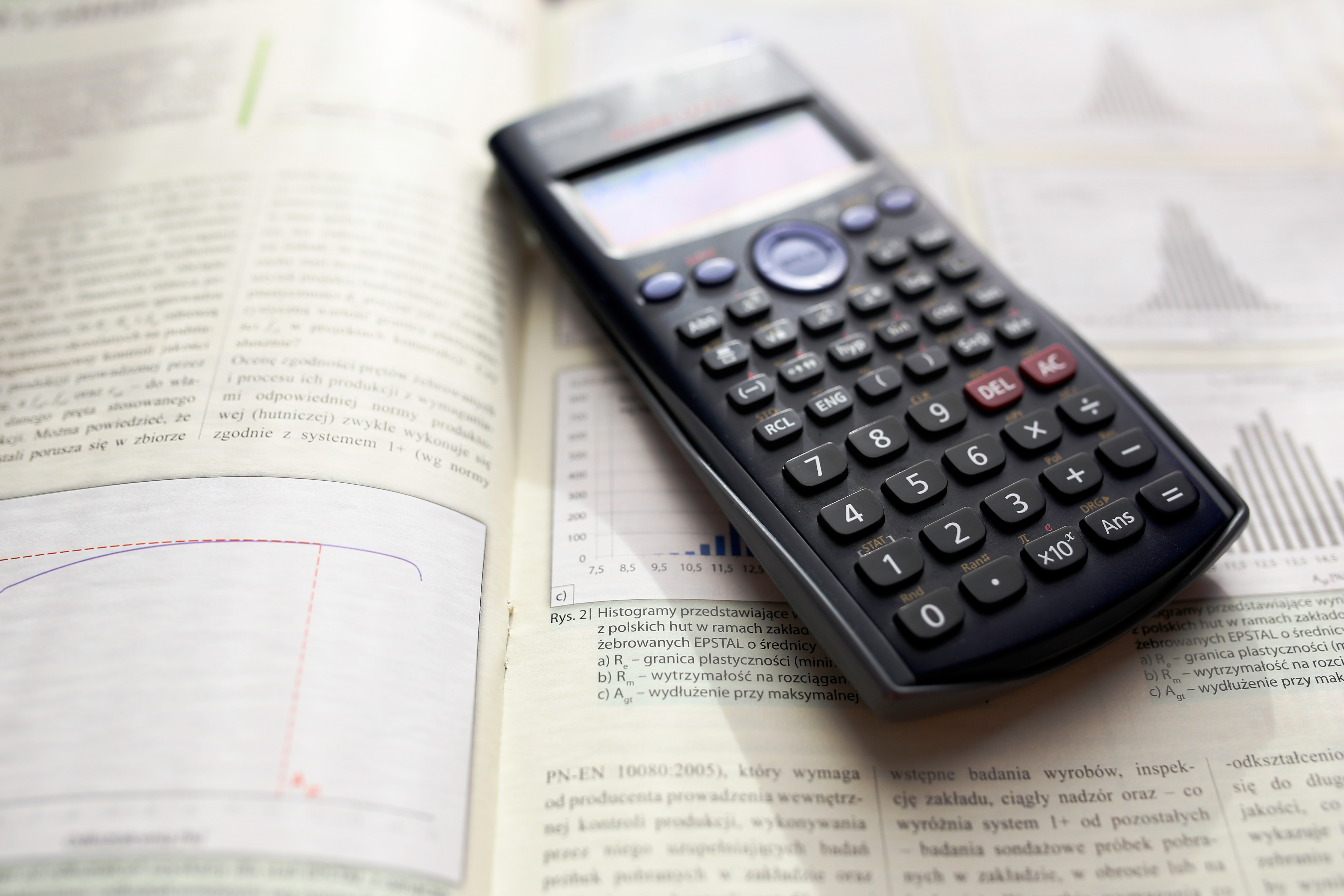# Solution to problems – class 9 – Set 1 Q 28

Last updated on June 17th, 2019 at 09:15 am

## Problem Statement

A bullet is moving at a speed of 350 m/s when it embeds into a lump of moist clay. The bullet penetrates for a distance of 0.05 m. Determine the acceleration of the bullet while moving into the clay. (Assume a uniform acceleration.)

## Solution to physics problem

Initial velocity of the bullet U=350 m/s
Final velocity of the bullet = 0
Distance travelled to acquire the final velocity =S=0.05 m

To find out the acceleration (here it is negative acceleration or retardation actually), we will use the equation of retardation
V2 = U2 – 2 a S
As V = 0, the equation becomes: U2 = 2 a S
Which gives retardation a = U2 / (2 S) = 3502/(2 x 0.05) =1225000 m/s2

So the answer would be: retardation =1225000 m/s2

Alternate way:
Here we will use the general equation of acceleration.
V2 = U2 + 2 a S
As V = 0, the equation becomes: U2 = – 2 a S
Which gives acceleration a = – U2 / (2 S) = – 3502/(2 x 0.05) =- 1225000 m/s2
So the answer would be: acceleration =- 1225000 m/s2